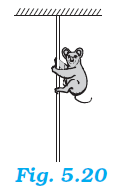## Pages

### Laws of motion NCERT Solutions Class 11 Physics - Solved Exercise Question 5.33

Question 5.33:
A monkey of mass 40 kg climbs on a rope (Fig. 5.20) which can stand a maximum tension of 600 N. In which of the following cases will the rope break: the monkey
(a) climbs up with an acceleration of 6 m s–2
(b) climbs down with an acceleration of 4 m s–2
(c) climbs up with a uniform speed of 5 m s–1
(d) falls down the rope nearly freely under gravity?
(Ignore the mass of the rope).Solution:
Case (a)
Mass of the monkey, m = 40 kg
Acceleration due to gravity, g = 10 m/s
Maximum tension that the rope can bear, Tmax = 600 N
Acceleration of the monkey, a = 6 m/s2 upward
Using Newton’s second law of motion, we can write the equation of motion as:
Tmg = ma
T = m(g + a)
= 40 (10 + 6)
= 640 N
Since T > Tmax, the rope will break in this case.

Case (b)
Acceleration of the monkey, a = 4 m/s2 downward
Using Newton’s second law of motion, we can write the equation of motion as:
mg – T = ma
T = m (g – a)
= 40(10 – 4)
= 240 N
Since T < Tmax, the rope will not break in this case.

Case (c)
The monkey is climbing with a uniform speed of 5 m/s. Therefore, its acceleration is zero, i.e., a = 0.
Using Newton’s second law of motion, we can write the equation of motion as:
Tmg = ma
Tmg = 0
T = mg
= 40 × 10
= 400 N
Since T < Tmax, the rope will not break in this case.

Case (d)
When the monkey falls freely under gravity, its will acceleration become equal to the acceleration due to gravity, i.e., a = g
Using Newton’s second law of motion, we can write the equation of motion as:
mg – T = mg
T = m(gg) = 0
Since T < Tmax, the rope will not break in this case.